# Program to check CoPrime Numbers Program in Java

In this tutorial you will learn about the Program to check CoPrime Numbers Program in Java and its application with practical example.

In this tutorial, we will learn to create a Java Program to check CoPrime Numbers Program in Java

## Prerequisites

Before starting with this tutorial, we assume that you are best aware of the following Java programming concepts:

• Java Operators.
• Basic Input and Output function in Java.
• Class and Object in Java.
• Basic Java programming.
• If-else statements in Java.
• For loop in Java.

## What is Co-prime Number.

The numbers are said to be co-prime, if their GCD is “1”
So 13,14 and 15 are co-prime numbers.

## Program to check CoPrime Numbers Program in Java

In this program we would find the given two number are co-prime or not first will take the input from the user and check they arr coprime or not. let have a look at the code

## Output

Co-prime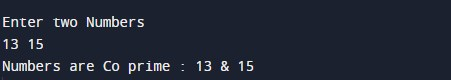Not a Co-prime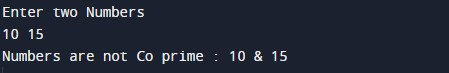In the above program, we have first declared and initialized a set variables required in the program.

• Num1,num2 = it will hold entered numbers
• a=  for iteration.
• c= for count.

After declaring variables we take two values from user.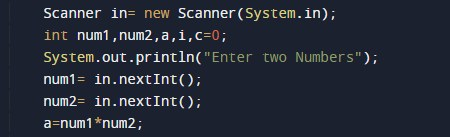Co-prime numbers are the numbers whose common factor is  1 lets look at the example.

after that we find factors of given number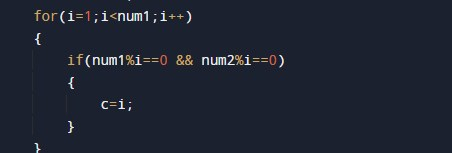if number has common factor 1 then they are co-prime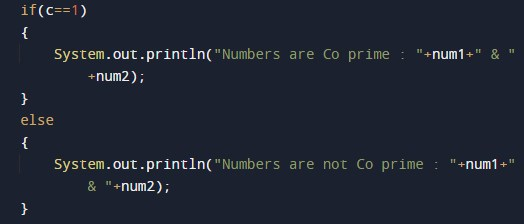if not they are not co-prime numbers.

In this tutorial we have learn about the Program to check CoPrime Numbers Program in Java and its application with practical example. I hope you will like this tutorial.